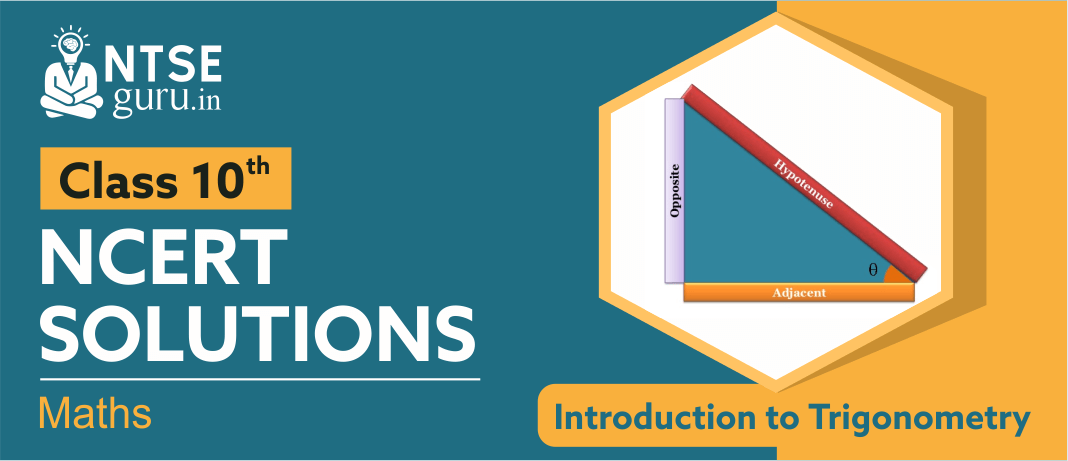# NCERT Solutions For Class 10 Maths Chapter 8Trigonometry is the study of triangles and the lengths and angles of their sides. As one of the most important fields of mathematics, particularly for careers that are built around calculating angles, a working knowledge of trigonometry and its uses is important for students of all ages. NCERT Solutions for Class 10 Maths Chapter 8, ‘Introduction to Trigonometry’ introduces students with Trigonometric ratios of some Specific Angles,Complementary Angles and Trigonometric Identities.

## Introduction to Trigonometry - Class 10 NCERT Solutions

Trigonometry developed from a need to compute angles and distances in such fields as astronomy, mapmaking, surveying, and artillery range finding. Problems involving angles and distances in one plane are covered in plane trigonometry.
Given below are ‘Introduction to Trigonometry - Class 10 NCERT Solutions’ for your reference.

In addition to the above solutions, students are also advised to follow sample papers, previous years solved papers and practice sets. All this material can be accessed from our website ntseguru.in or from our free mobile app NTSE Guru.